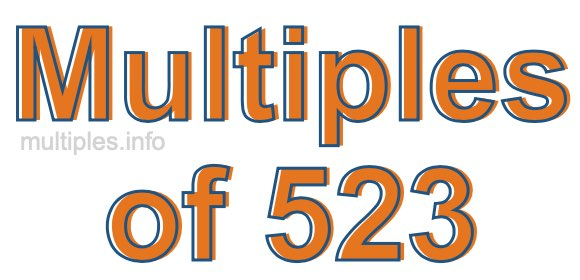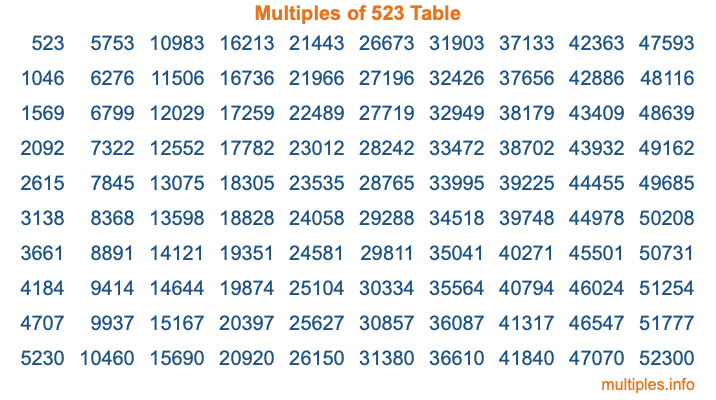Multiples of 523Welcome to the Multiples of 523 page. Here we will first teach you everything you will ever need to know about the multiples of 523, and then give you a study guide summary of everything we taught you to make sure you remember it all. Use this page to look up facts and learn information about the multiples of 523. This page will make you a multiples of five hundred twenty-three expert!

Definition of Multiples of 523
Multiples of 523 are all the numbers that when divided by 523 equal an integer. Each of the multiples of 523 are called a multiple. A multiple of 523 is created by multiplying 523 by an integer.

Therefore, to create a list of multiples of 523, you start with 1 multiplied by 523, then 2 multiplied by 523, then 3 multiplied by 523, and so on for as long as you want. Thus, the list of the first five multiples of 523 is 523, 1046, 1569, 2092, and 2615. To see a larger list of multiples of 523, see the printable image of Multiples of 523 further down on this page. We also have a category where you can choose any nth multiple of 523.

Multiples of 523 Checker
The Multiples of 523 Checker below checks to see if any number of your choice is a multiple of 523. In other words, it checks to see if there is any number (integer) that when multiplied by 523 will equal your number. To do that, we divide your number by 523. If the the quotient is an integer, then your number is a multiple of 523.

Is  a multiple of 523?

Least Common Multiple of 523 and ...
A Least Common Multiple (LCM) is the lowest multiple that two or more numbers have in common. This is also called the smallest common multiple or lowest common multiple and is useful to know when you are adding our subtracting fractions. Enter one or more numbers below (523 is already entered) to find the LCM.

Check out our LCM Calculator if you need more details about the Least Common Multiple or if you need the LCM for different numbers for adding and subtraction fractions.

nth Multiple of 523
As we stated above, 523 is the first multiple of 523, 1046 is the second multiple of 523, 1569 is the third multiple of 523, and so on. Enter a number below to find the nth multiple of 523.

th multiple of 523

Multiples of 523 vs Factors of 523
523 is a multiple of 523 and a factor of 523, but that is where the similarities end. All postive multiples of 523 are 523 or greater than 523. All positive factors of 523 are 523 or less than 523.

Below is the beginning list of multiples of 523 and the factors of 523 so you can compare:

Multiples of 523: 523, 1046, 1569, 2092, 2615, etc.

Factors of 523: 1, 523

As you can see, the multiples of 523 are all the numbers that you can divide by 523 to get a whole number. The factors of 523, on the other hand, are all the whole numbers that you can multiply by another whole number to get 523.

It's also interesting to note that if a number (x) is a factor of 523, then 523 will also be a multiple of that number (x).

Multiples of 523 vs Divisors of 523
The divisors of 523 are all the integers that 523 can be divided by evenly. Below is a list of the divisors of 523.

Divisors of 523: 1, 523

The interesting thing to note here is that if you take any multiple of 523 and divide it by a divisor of 523, you will see that the quotient is an integer.

Multiples of 523 Table
Below is an image of the first 100 multiples of 523 in a table. The table is in chronological order, column by column. The first column has the first ten multiples of 523, the second column has the next ten multiples of 523, and so on.The Multiples of 523 Table is also referred to as the 523 Times Table or Times Table of 523. You are welcome to print out our table for your studies.

Negative Multiples of 523
Although not often discussed or needed in math, it is worth mentioning that you can make a list of negative multiples of 523 by multiplying 523 by -1, then by -2, then by -3, and so on, to get the following list of negative multiples of 523:

-523, -1046, -1569, -2092, -2615, etc.

Multiples of 523 Summary
Below is a summary of important Multiples of 523 facts that we have discussed on this page. To retain the knowledge on this page, we recommend that you read through the summary and explain to yourself or a study partner why they hold true.

There are an infinite number of multiples of 523.

A multiple of 523 divided by 523 will equal a whole number.

523 divided by a factor of 523 equals a divisor of 523.

The nth multiple of 523 is n times 523.

The largest factor of 523 is equal to the first positive multiple of 523.

523 is a multiple of every factor of 523.

523 is a multiple of 523.

A multiple of 523 divided by a divisor of 523 equals an integer.

523 divided by a divisor of 523 equals a factor of 523.

Any integer times 523 will equal a multiple of 523.

Multiples of a Number
Here you can get the multiples of another number, all with the same attention to detail as we did for multiples of 523 on this page.

Multiples of
Multiples of 524
Did you find our page about multiples of five hundred twenty-three educational? Do you want more knowledge? Check out the multiples of the next number on our list!

Copyright  |   Privacy Policy  |   Disclaimer  |   Contact